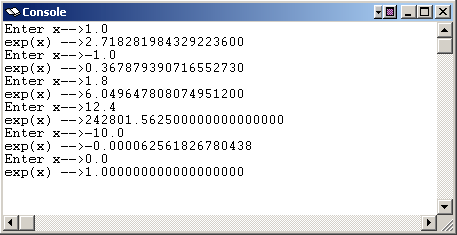created 05/22/2004

# Chapter 32 Programming Exercises

NOTE: If you have read the chapters on subroutine linkage, write each exercise as a main program calling a subroutine. Use either the stack-based linkage or the frame-based linkage convention.

In the Settings menu of SPIM set Bare Machine OFF, Allow Pseudo Instructions ON, Load Trap File ON, Delayed Branches OFF, Delayed Loads OFF, Mapped IO ON, Quiet OFF.

Run the programs by clicking SimulatorGo and then OK in the pop-up panel.

## *Exercise 1 — exp(x)

Write a program that computes exp(x) by using a Taylor series:

```exp(x) = 1 + x + x2/2 + x3/3! + x4/4! + ...
```

The user enters x. exp(x) is ex, where e is the base of the natural logarithms, 2.718281828... You don't need to worry about the math behind this. Just compute a sum of terms. Each term in the sum looks like:

```xn/n!
```

Designate a register to hold the current term. Initialize it to 1.0 (the zeroeth term). Term one is calculated by multiplying:

```term*x/1
```

Term one is the value x. Term two is calculated by multiplying:

```term*x/2
```

Term two is the value x2/2. Term three is calculated by multiplying:

```term*x/3
```

Term three is the value x3/3!. Term four is calculated by multiplying:

```term*x/4
```

... and so on. In general, after you have added term (n-1)to the sum, calculate term n by multiplying:

```term *x/n
```

Keep doing this in a loop until the term becomes very small. Here are some example runs (with SPIM using single precision). As usual, only the first seven digits printed have any significance.It might be helpful to first write this program in Java or C to verify your design, and then to proceed with the version in assembly language.

## *Exercise 2 — pi

Various infinite series for pi have been discovered. The first such series was

```pi = 4( 1 - 1/3 + 1/5 - 1/7 + 1/9 - . . .)
```

This series is of little practical value because enormous numbers of terms are required to achieve good approximations (Acton, Calculus, 1999). However, it does provide good programming practice.

Write a SPIM program that writes out the sum of the first 1000, 2000, 3000, ... , 10000 terms of the series.

As with the previous program, write a version of this program in C or Java first to check that your design is correct.